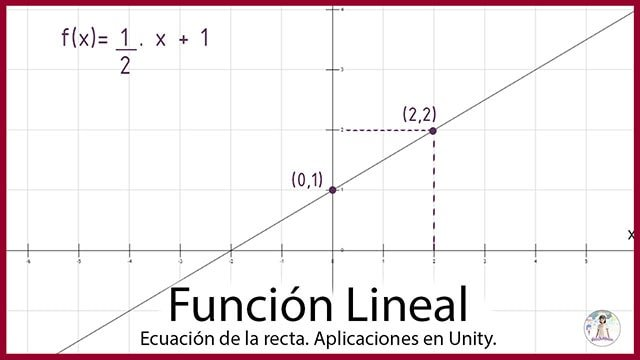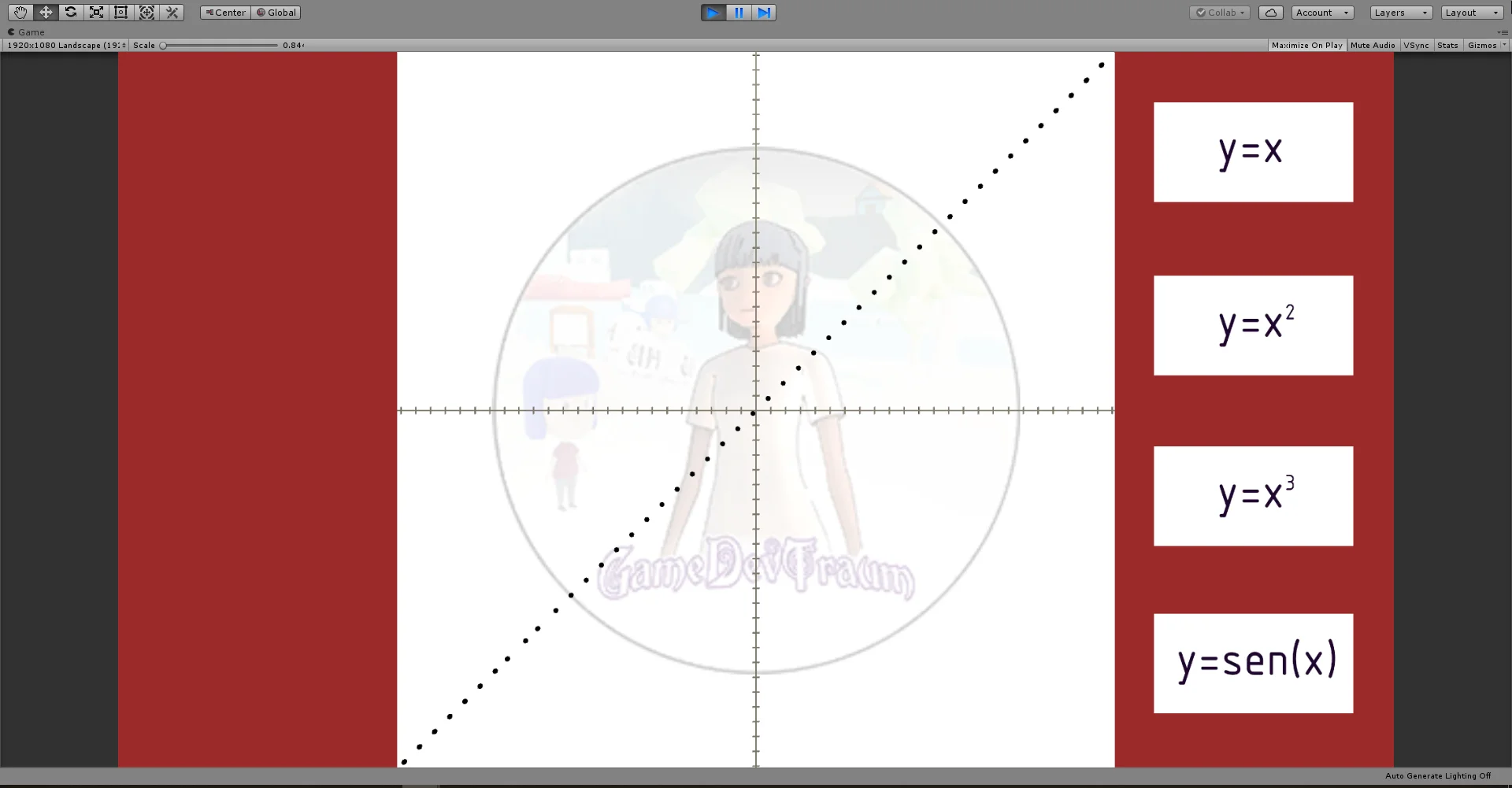# Linear function. Characteristics, graph and examples of use in Unity

## Introduction

In this article we are going to see what a linear function is, its mathematical expression, its characteristics, how to graph it in the Cartesian plane and what it can be useful for in the development of videogames with examples in Unity.

## Mathematical expression of a linear function

A linear function is a polynomial function whose expression is:

f (X) = a . X + b

It is commonly read “f of x”, being X the independent variable, a and b constant real numbers.

Analyzing the expression we see that given any value of X, we first multiply it by a and then add b. The result of all that operation will be the value of f (X).

## Characteristics of a linear function

### Domain

The domain is the range of allowable values for the independent variable, commonly referred to as X.

In the case of the linear function, the domain is the set of real numbers.

In other words we can choose any value of X belonging to the set of real numbers and we will find its corresponding value f (X).

### Graph in the Cartesian plane of a linear function

The graph of f (X) in the Cartesian plane is a straight line. We can easily draw it by finding two points of the function and then using a ruler, draw the line that joins the two points.

We can easily find one of these points by considering X = 0.

The second point can be found by choosing a different value for X and making calculations, for example for the function in figure 1, if we consider X = 2, the result of f (X) is equal to 2.

### Ordinate to the origin

It is the value of the function when X = 0, graphically it is the point where the function cuts off the vertical axis known as the ordinate axis. This point is known as the ordinate to the origin.

In the graph of figure 1 we see that the ordinate to the origin is the point (0,1).

### Abscissa of the origin

Análogamente al caso anterior, la abscisa al origen es el punto en el cual la función corta el eje horizontal o eje de abscisas. En este punto Y = 0.

Una función lineal podría no tener abscisa al origen si se trata de una recta paralela al eje x y desplazada.

En la gráfica de la figura 1 vemos que la abscisa al origen es el punto (-2,0).

### Slope of a linear function

The term that multiplies X is known as slope and is the one that establishes how much the function changes when the variable X changes by one unit.

If we only have the graph of a linear function, we can calculate the slope as the tangent of the angle that forms the line with the horizontal axis. We can also find the slope using the Pythagorean Theorem.

### Parallel lines

Two straight lines are parallel if they have the same slope.

Example of two parallel lines:

f (X) = 2 . X – 1

g (X) = 2 . X + 3

### Perpendicular lines

Two straight lines are perpendicular if the slope of one of them is equal to inverting the slope of the other and multiplying it by -1.

Example of two perpendicular lines:

f (X) = 3 . X + 2

g (X) = – ( 1/3 ) . X + 5

## Some examples in Unity of linear function

The linear function is one of the most useful mathematical functions and its field of application is very varied. Let’s give some examples of possible applications.

### Represent trajectories

The most basic thing I can think of is for objects to move in a straight path, which can be described as a linear function.

For example in figure 2 we see a scene in Unity in which the spheres are describing a trajectory that is described as their position in x is equal to their position in y.Fig. 2: The spheres are advancing in the positive direction of the X-axis, their height Y is determined by the calculation of function y = x.

The same concept and with different combinations of planes can be applied to other cases such as vehicle movements, projectile trajectories, etc.

In the last video of my Unity fundamental series, I use a linear function for the movement of the camera, which advances inside the tunnel.

### Relate parameters linearly

Let’s suppose that we have any two parameters, for example the position of the player on the X axis and the rotation of a mechanism with respect to one of its axes.

Let’s also say that we would like these parameters to be connected in some way, that is to say that the rotation of the mechanism will depend on the position of the player.

We can then establish a linear relationship between these two parameters, for example we could say that the rotation of the mechanism with respect to its z-axis is equal to half the value of the player’s position on the x-axis plus five units.

With this we can relate these two parameters through a linear function.

### Implement linear magnitudes in our game

In physics there are many linear magnitudes that we might be interested in implementing in our game. An example of this can be the uniform rectilinear movement, in which we have a direction of movement established and the position will be defined by a linear function in which the slope of the line is the velocity of the object and the ordinate to the origin is the initial position.

In the case of Ohm’s Law for example, if we have a circuit with a source connected to a resistance, the circulating current is equal to the voltage of the source divided the resistance, this is nothing other than a linear function with the form i = v / R.

The potential gravitational energy of an object is a linear function of its height above ground.

There are several physical phenomena that are characterized by linear behavior and that we might be interested in implementing.

## Conclusion

The linear function is one of the most basic functions but also one of the most useful.

The graph in the Cartesian plane corresponds to a line in which we can identify a slope and an ordinate to the origin, point in which the line cuts to the vertical axis or abscissa.

We can use it to establish linear relationships between different parameters of our game and represent physical phenomena of linear character.